# Calibration-Free Return Loss Measurement

Return loss in a wired or wireless transmitter is the ratio of transmitted power to reflected power when power is being delivered to a load. It is a critical parameter that describes transmission efficiency and can be used as an indicator that excessive power is being reflected from a load back to the source. In a wireless transmitter with poor antenna return loss, a high level of reflected power can damage the power amplifier that is driving the antenna.

This article presents a novel method to accurately measure return loss in a wired or wireless transmitter in real time over a broad frequency range (2 GHz to 26 GHz) without the need for any system calibration.

### Circuit Operation

Figure 1 shows the complete circuit where return loss is being measured between an RF amplifier and its load; the load will be an antenna in a practical wireless system. Incident and reflected power are sensed using a broadband directional coupler with a frequency range from 2 GHz to 26.5 GHz.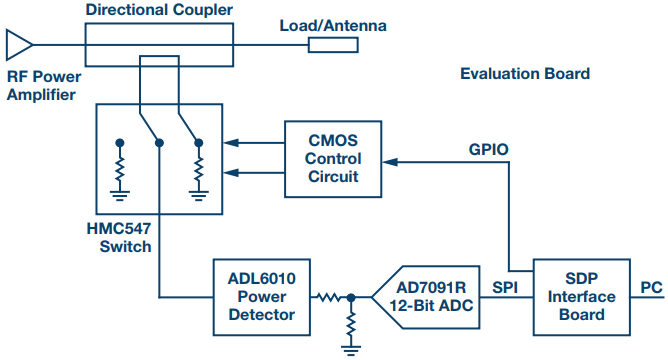Figure 1. Functional block diagram.

An RF switch is used to alternately connect to the coupled and isolated port of the directional coupler. The control lines on the RF switch are periodically toggled using negative logic CMOS control circuitry. An absorptive RF switch is used to ensure that both ports of the directional coupler are always terminated with 50 Ω. The coupled RF signal output from the switch then drives the input of the ADL6010, a broadband microwave detector that operates from 500 MHz to 43.5 GHz.

The AD7091R 12-bit ADC samples the output of the ADL6010 RF detector at a rate of 1 MSPS. This converts the analog output voltage of the detector to a digital code. Using the forward and reverse codes, the return loss can be calculated without any need for system calibration.

### Calibration-Free Return Loss Measurement and Calculation

Figure 2 shows the transfer function of the ADL6010 integrated microwave diode detector in terms of dc output voltage vs. ac input voltage. This detector has a 45 dB detection range and operates from 500 MHz to 43.5 GHz.Figure 2. ADL6010 integrated diode detector transfer function in VOUT vs. VIN.

This device has a so-called linear in V/V transfer function. This means that the dc output voltage is directly proportional to the input ac voltage when the input power is within its 45 dB linear operating region (approximately –30 dBm to +15 dBm or 7.1 mV rms to 1.3 V rms). Unlike a traditional Schottky diode-based detector, there is no square law region of operation. This results in a transfer function that can be modeled using a simple linear equation:where m is the slope in V/V and b is the y-axis intercept.

Rewriting this equation in terms of power in dBm, we get:where R is the system impedance, usually 50 Ω.

The output response of the power detector will vary in temperature, frequency, and from device-to-device. So the values for m and b in the previous equation will vary and must generally be determined using calibration.

To back calculate the input power to the detector using the output voltage, calibration is required at each frequency and on each device. However, in the circuit shown in Figure 1, we are only trying to measure return loss. Since the forward and reverse power are being measured by the same detector, it can be proven that the return loss can be calculated using the following equation:where VF and VR are the measured output voltages from the detector when the RF switch connects the directional coupler to the forward and reverse coupled ports of the directional coupler respectively. This equation holds true when the y-intercept (b) is close to zero, which is the case for this particular detector (see Figure 2).

This equation is significant because the m and b terms are no longer present. This means that precise return loss can be measured without any system calibration.

In practice, it is the codes from the ADC in Figure 1 that are used to perform the calculation. So the final equation becomes: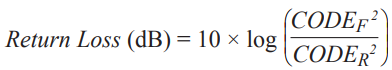Again, because the ADC has a y-axis intercept close to 0, we are not required to perform any calibration of the ADC to measure precise return loss.

Reflection coefficient is even easier to calculate because the requirement for a log(x) calculation falls away. This yields the equation: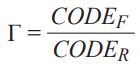and VSWR can be calculated using the equation: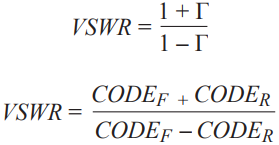### Test Results

Figure 3 shows measured return loss vs. input power at 2 GHz when a return loss of 20 dB is present.Figure 3. Return loss measurement results.

To mimic an antenna with a 20 dB return loss, a 9 dB attenuator with an open circuited output was connected to the directional coupler’s output. Ideally this should result in 18 dB of return loss. However, when the effect of cable loss, connections, and coupler insertion loss are factored in, the true return loss of this test load was determined to be approximately 20 dB.

From the graph in Figure 3, we see that for input powers from 0 dBm to +25 dBm, the measured return loss remains close to 20 dB. Above and below these levels, the measured return loss degrades significantly. On the high side (this is due to the power at the detector’s input exceeding its saturation point of +15 dBm) the +27 dBm power through the directional coupler appears as +15 dBm at the RF detector due to the coupling factor and the insertion loss of the switch.

On the low side (input powers below 0 dBm), the error is caused by the detector’s sensitivity. A 0 dBm input power is reflected back from the load at –20 dBm. This level drops by approximately 12 dB through the coupler and RF switch, arriving at the detector as a power level of approximately –32 dBm, which is below the input sensitivity of the ADL6010 detector.

### Selecting a Directional Coupler

Every directional coupler has both a coupled and an isolated port, as shown in Figure 4. The forward coupled signal appears on the coupled output and the signal reflected from the load is coupled to the isolated port. On most directional couplers, the isolated port is terminated with a permanent, unremovable 50 Ω termination. For this application, the Marki Microwave C10-0226 was chosen for a number of reasons. The device has a broad operating frequency range (2 GHz to 26 GHz) that covers a significant portion of the ADL6010 detector’s range. Over this range, it has an input return loss and directivity of 20 dB or better. In order to measure a return loss of 20 dB on a load, the directivity and input return loss of the coupler itself must at least exceed this number.Figure 4. RF directional coupler.

The isolated port on the C10-2226 coupler does not have a fixed termination. Instead the user can attach a 50 Ω SMA load for normal operation. However, in this case we take advantage of this feature and use the isolated port to measure the reverse power. So we effectively have a single device that can sense incident and reflected power.

The coupling factor of the coupler is 10 dB. Coupling factor has a significant impact on the circuit level planning, shown in Figure 5. In order to optimize the detection range of the circuit, the maximum power at the antenna port should map to the maximum input power of the detector. So in this example, the 10 dB coupling factor (along with 2 dB of insertion loss for the RF switch) and the detector’s maximum input power of +15 dBm set the maximum power at the antenna port to be +27 dBm. If higher output power is desired, then a directional coupler with a higher coupling factor can be used. This would have the advantage of slightly lower coupler insertion loss. Alternatively, additional attenuation could be inserted between the output of the switch and the input of the detector.Figure 5. Circuit level planning.

In a practical circuit, a surface-mount directional coupler may be preferable. These devices will tend to have similar insertion loss to the connectorized coupler that is used here. But their bandwidth, directivity, and isolation will tend to not be as good.

### RF Switch Selection Considerations

The HMC547LC3 switch is used in this application. This is a single-pole, double throw, nonreflective switch with an input frequency range from dc to 28 GHz with a high speed switching time of 6 ns.

The nonreflective characteristic of this switch is critical to the correct operation of the overall circuit. Without the dummy 50 Ω load that the switch presents at its inputs when they are not connected, the directional coupler would not be properly terminated.

The insertion loss of the switch is not critical in this application. The insertion loss of the switch effectively increases the coupling factor of the directional coupler. Also, since forward and reverse power are routed through the same path, any variations vs. temperature and frequency cancel out. The switch, along with the coupler, limits the operation of the circuit to a maximum of 28 GHz. To operate the circuit up to the maximum input frequency of the ADL6010 detector, a higher frequency switch would have to be used.

### ADC Selection Considerations

The AD7091R is a 12-bit successive approximation register SAR ADC. This is a low power consumption ADC of typically 349 μA at a throughput rate of up to 1 MSPS. Lower throughput rates can be used, which in turn reduces power consumption.

This ADC was chosen primarily because it has enough resolution to detect the output voltage from the ADL6010 detector over its full input range. The so-called linear in V/V transfer function of the detector (shown in Figure 2) means that the incremental output slope in V/dB decreases with input power. Therefore a 12-bit ADC was selected so that input power changes that are less than 1 dB can be resolved even when the input power is at the bottom end of the detector’s input range.

In the practical implementation of this circuit, each digital code from the ADC is transferred over 3-wire SPI interface to a PC. A software routine on the PC then calculates and displays the return loss.

The time taken to measure both forward and reverse coupled signals, and calculate return loss is approximately 1.4 ms—500 forward and 500 reverse samples are collected on each cycle. The large number of samples provides averaging, which is necessary in applications where the signal has a fast changing envelope. In addition, some low-pass filtering (a simple R to C circuit without any buffering) was placed between the output of the detector and the input of the ADC to provide additional averaging.

The software program requires approximately 400 μs to perform the switching operation between forward and reverse sampling. This results in an update rate of 1.4 ms.

Alternative sampling schemes could also be used where faster switching is available (the switching rate in the previous example is limited by the control software, not by the switch itself).

### RF Power Measurement

The focus up until now has been on measuring return loss without the need for any calibration. With the addition of a simple calibration routine, the circuit can also be used to accurately measure transmitted power. Figure 6 shows where input power was swept at 2 GHz and power was back calculated using the code from the ADC along with the values for m and b, which are acquired during calibration.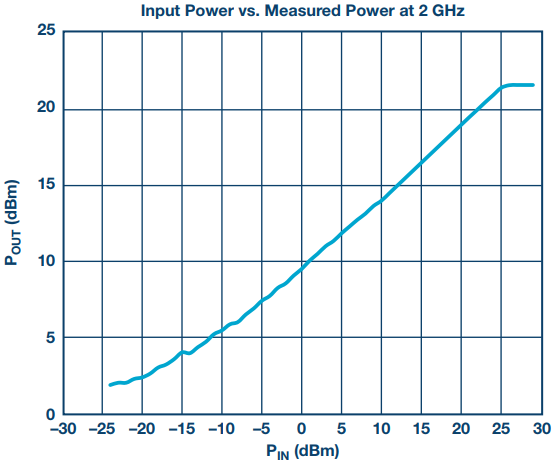Figure 6. Measured power vs. applied power when the circuit is used to measure absolute RF input power.

### Conclusion

The circuit described provides a convenient solution for measuring precise return loss where performing calibration is either not possible or undesirable. It has an absolute power detection range of 45 dB. This allows for measurement of return loss of up to 20 dB over an RF power range of 25 dB. The absolute power range can be scaled upwards from its minimum range of 0 dBm to +25 dBm.

While the RF detector used has an input frequency range from 500 MHz to 43.5 GHz, the frequency range of the circuit will typically be limited either by the RF switch or directional coupler that is used, particularly if a surface-mount coupler is used.

The circuit described has been implemented on a single PCB (except for the directional coupler) and it is available at Analog Devices. For further details, go to www.analog.com/CN0387.

### 参考資料

1CN-0387–Calibration-Free Return Loss Measurement System. Analog Devices.

### 謝辞

1. Patrick Walsh, Joel Dobler, Vincent Heffernan—Analog Devices, Applications.

2. Mike O’Shea—Analog Devices, Design.